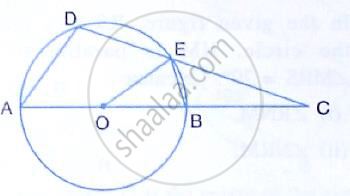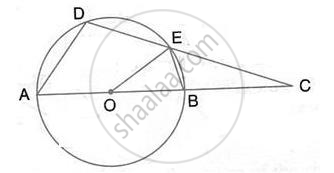Share

In the Figure, O is the Centre of the Circle, ∠Aoe = 150°, ∠Dao = 51°. Calculate the Sizes of the Angles Ceb and Oce. - ICSE Class 10 - Mathematics

ConceptArc and Chord Properties - the Angle that an Arc of a Circle Subtends at the Center is Double that Which It Subtends at Any Point on the Remaining Part of the Circle

Question

In the figure, O is the centre of the circle, ∠AOE = 150°, ∠DAO = 51°. Calculate the sizes of the angles CEB and OCE.Solution∠ADE = 1/2Re flex (∠AOE) = 1/2 (360° -150° ) = 105°

(Angle at the center is double the angle ate the circumference subtended by the same chord)
∠DAB + ∠BED = 180°
(pair of opposite angles in a cyclic quadrilateral are supplementary)
⇒ ∠BED =180° - 51° = 129°

∴ ∠CEB = 180° - BED  (straight line)

=180° -129° = 51°
Also, by angle sum property of ∆ADC,
∠OCE =180° - 51° -105° = 24°

Is there an error in this question or solution?

Video TutorialsVIEW ALL 

Solution In the Figure, O is the Centre of the Circle, ∠Aoe = 150°, ∠Dao = 51°. Calculate the Sizes of the Angles Ceb and Oce. Concept: Arc and Chord Properties - the Angle that an Arc of a Circle Subtends at the Center is Double that Which It Subtends at Any Point on the Remaining Part of the Circle.
S